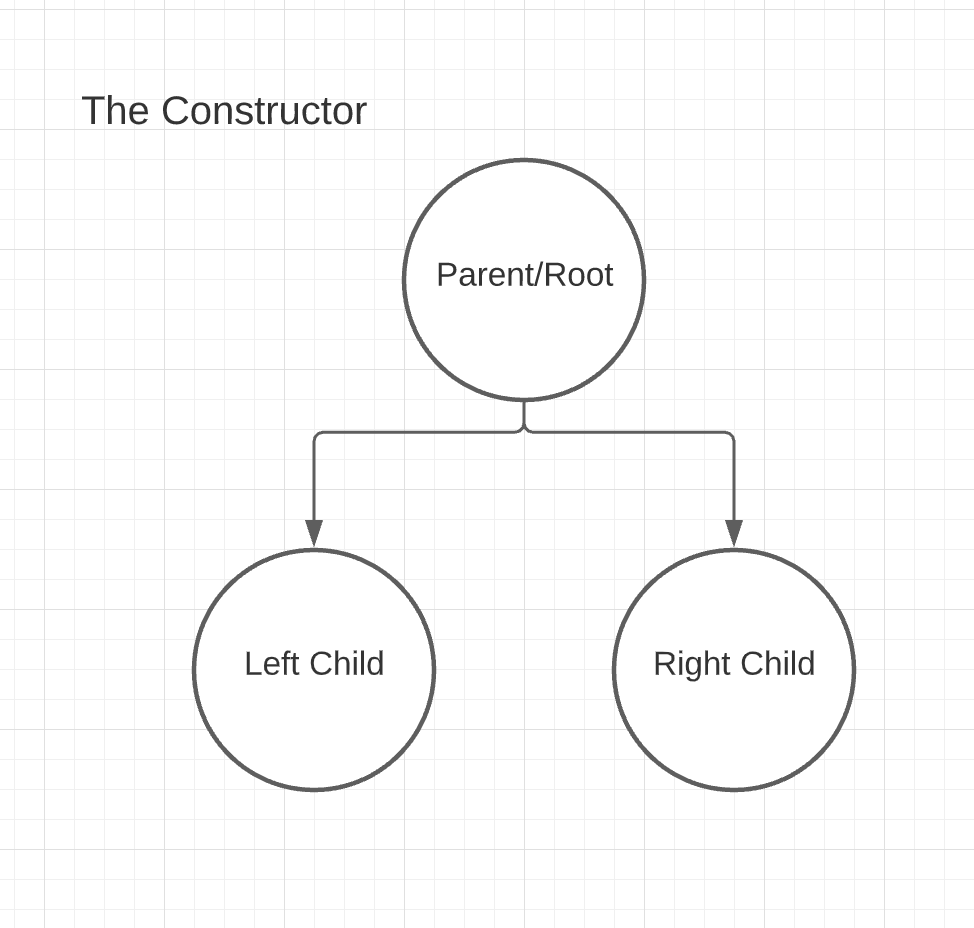# Binary Search Tree Constructionsource

# Why?

Binary Search Tree (BST) is a data structure that provides us the fast lookup times, insertion and removal of the nodes. The data structure is especially efficient when it comes to fast lookup and implementation of dynamic sets of items or lookup tables. Some of the huge tech companies use this data structure to create what we take for granted for example: Facebook is able to search through billions of users’ data quick or Google search engines are able to populate suggestions as you start typing.

This data structure was quite a mystery to me because I wanted to solve Leetcode questions around it but never had the understanding yet to. However, I realized it really comes down to how familiar the data structure works in terms of the three main fundamental functions: insert, search, and (God forbid) remove.

At first glance, the easiest part of solving BST questions are the add and search functions. Because there are usually less code involved and you are not replacing anything. However, removing is a different story. After removing the value, you are essentially going to replace the parent value with the minimum value from the further right branch of the BST even with remove, the goal of the article is to give the reader an overview of how the three fundamental functions are implemented and explain the logic behind the code.

# Constructordemonstration of the constructor structure

# Overview and Time/Space Complexity

First there are two common methods of approaching the BST methods . First is the recursion method and the second one is the iterative approach.

Recursion and iterative approach in time complexity perspective is the arguably the same.

Assuming the binary search tree is balanced, the time complexity will be on average O(log(N)) where N is the number of nodes in the tree that is being traversed. However, if the binary search tree is unbalanced where it is impossible and that the algorithm has to traverse every height of the tree (which is the worst case for this algorithm), then the time complexity will be O(N) where N is the number of nodes in the tree. This is because the algorithm will have to go through every nodes of the tree instead of ignoring the parts of the tree that don’t need to be searched.

However, the iterative approach is arguably more efficient because the recursive takes up space in our the insert method O(log(N)) where N is the number of nodes in the tree on the average case and O(N) where N is the number of nodes in the tree on the worst case just in case the tree that we are dealing with is not balanced.

Recursion:

Iteration:

Recursive:

Iteration:

# Remove

Recursive:

Iteration:

The iterative method’s logic should be a similar one to the recursive. Check it out

# Conclusion

Binary Search Tree definitely takes some time to take in. However, if you take the time to learn the core concepts which is Insert, Contains and Remove by heart, you will be off to tackle those Leetcode BST questions in no time!

# Reference

Check out Algoexpert.io and way to go Clement!

Hi, i’m a software engineer based in NYC!